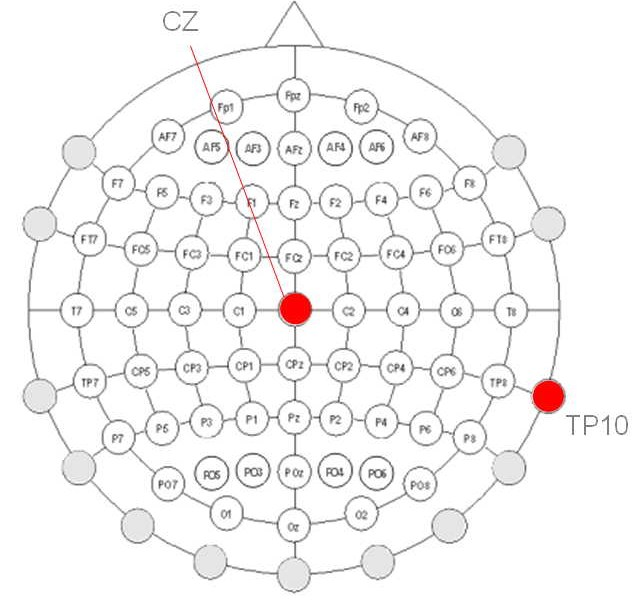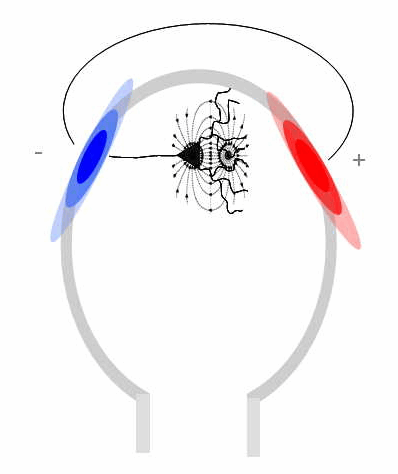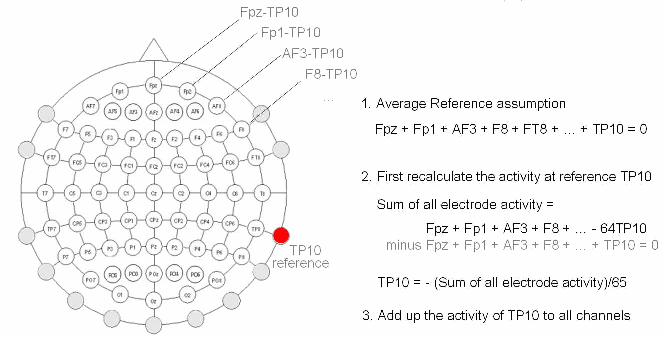# Background on EEG references

Refer to the re-referencing tutorial for learning how to reference EEG data in EEGLAB.

## What is re-referencing, and why re-reference?

The reference electrode used in recording EEG data is usually termed the ‘common’ reference for the data – if all the channels use this same reference. Typical recording references in EEG recording are one mastoid (for example, TP10 in the 10-20 System, the electrode colored red in the picture below), linked mastoids (usually, digitally-linked mastoids, computed post hoc), the vertex electrode (Cz), single or linked earlobes, or the nose tip. Systems with active electrodes (e.g., BIOSEMI Active Two) may record data reference-free. In this case, a reference be must be chosen post hoc during data import. Failing to do so may leave 40 dB of unnecessary noise in the data.

There is no ‘best’ common reference site. Some researchers claim that non-scalp references (earlobes, nose) introduce more noise than a scalp channel reference though this has not been proven to our knowledge. If the data have been recorded with a given reference, they can usually be re-referenced (inside or outside EEGLAB) to any other reference channel or channel combination.Converting data, before analysis, from fixed or common reference (for example, from a common earlobe or other channel reference) to ‘average reference’ is advocated by some researchers, particularly when the electrode montage covers nearly the whole head (as for some high-density recording systems).

The advantage of average reference rests on the fact that outward positive and negative currents, summed across an entire (electrically isolated) sphere, will sum to 0 (by Ohm’s law). For example, in the figure below, a tangentially-oriented electrical source is associated with a positive inward current to the left (here, blue) and an opposing outward negative current to the right (red). If the current passing through the base of the skull to the neck and body is assumed to be negligible (for instance, because of low conductance of the skull at the base of the brain), one may assume that the sum of the electric field values recorded at all (sufficiently dense and evenly distributed) scalp electrodes is always 0 (the average reference assumption).

The problem with this assumption is that true average reference data would require the distribution of electrodes to be even over the head. This is not usually the case, as researchers typically place more electrodes over certain scalp areas and fewer (if any) on the lower half of the head surface. As a consequence, an average reference result using one montage may not be directly comparable to an average reference result obtained using another montage.In the re-referencing tutorial, we detail the process of transforming data to ‘average reference’. Note that in this process, the implied activity time course at the former reference electrode may be calculated from the rest of the data (so the data acquires an additional channel - though not an additional degree of freedom). Also, note that if the data were recorded using nose tip or ear lobe electrodes, you should not include these reference electrodes when computing the average reference in (1) (below). Thus, in the tutorial example, the dividing factor (in (2)) would be 64 instead of 65. Note that in localizing sources using the EEGLAB DIPFIT plugin, ‘average reference’ will be used internally (without user input).

The choice of data reference does color (literally) the plotted results of the data analysis. For example, plots of mean alpha power over the scalp must have a minimum at the reference channel, even if there are alpha sources just below and oriented toward the reference channel. Of note, no (valid) reference can be said to be wrong. Instead, each reference can be said to give another view of the data. However, the nature of the reference needs to be considered when evaluating (or, particularly, comparing) EEG results with those of the literature.## Note on re-referencing and ICA decompositions

For ICA decomposition, in theory the selection of a reference is not so important. This is because changing the reference only amounts to making a linear transformation of the data (in mathematical terms, multiplying it by a fixed re-referencing matrix), a transformation to which ICA should be insensitive. In practice, we transform the data to average reference before running ICA, but the benefits of using this transformation have not been demonstrated.

We advise recording eye channels (conventionally four channels, two for vertical eye movement detection and two for horizontal eye movement detection) using the same reference as other channels instead of using bipolar montages. One can always recover the bipolar montage activity by subtracting the activities of the electrode pairs. We term these channels ‘peri-ocular EEG’ channels since what they record is not exclusively electro-oculographic (EOG) signals but also includes prefrontal EEG activities.

ICA can be used to decompose data from either average reference, common reference, or bipolar reference channels – or from more than one of these types at once. However, plotting single scalp maps requires that all channels use either the same common reference or the same average reference.